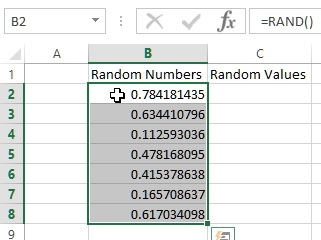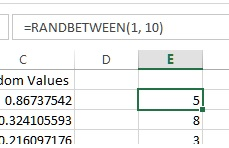# Random numbers in Excel

##### How to generate random numbers between two values in MS Excel?

In last post, we learnt about statistical functions of MS Excel. In this post, we shall learn how to generate random numbers in MS Excel.

### RAND function

RAND (Random) function is used to generate random numbers in MS Excel whose range will be between 0 to 1.

B2 cell has '=RAND()' formula that returns random value and when we copy the same formula till 8th row we get following results.Important: The default behavior of all RAND function is that it's value is re-calcuated everytime any cell on the sheet is calculated or a any cell value on the sheet is changed.

So when you change any value on the cell, the existing value of RAND function changes. To avoid this, we can copy the cell and paste it as Paste As Value as shown below.### RANDBETWEEN function

To get random number between two values, we can use RANDBETWEEN funciton. RANDBETWEEN accepts two parameters

• bottom - the lowest number
• top = the highest number
In below example E2 cell contains '=RANDBETWEEN(1, 10)' formula that would return random  number between 1 to 10.Important: The default behavior of all RANDBETWEEN function is that it's value is re-calcuated everytime any cell on the sheet is calculated or a any cell value on the sheet is changed.

Views: 2312 | Post Order: 38